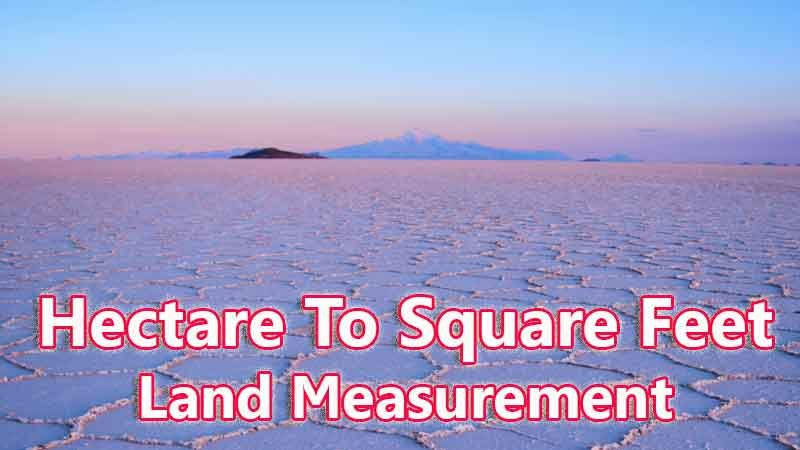# Hectare To Square Feet Converter

Hectare is a traditional land unit used to measure the land Area standardly. Here we are using the hectare to square feet unit value conversion. And hectare also comes under these land area units like Gonda, Kani, and square feet. In West Bengal, One hectare is directly equivalent to 107639 square feet.

Input Here

hectare

Output

square feet

1 hectare = 107639 square feet

## Measurement

Hectare means - a unit of land measurement. This tool provides great calculation over hectare to square feet, 1 hectare to square feet, 1 hectare in square feet, 1 hectare equal to and etc. To convert hectare to square feet, then multiply the unit value by 107639. Then, 1 hectare * 107639 = 107639 square feet. Hence, 1 hectare is equal to 107639 square feet.

square feet also comes under this land measurement unit and we are comparing values between hectare traditional unit with other side land measurement unit.

## Formula For hectare to square feet Conversion(hectare to square feet formula)

The base formula for this hectare to square feet converter is

square feet = hectare * 107639

## How many square feet in a hectare?

Step 1: To Convert 1 hectare to square feet

Step 2: Applying formula square feet = hectare * 107639, (i.e) multiply the unit value by 107639.

Step 3: Then, square feet = 1 * 107639 = 107639.

Step 4: Hence, 1 hectare is equal to 107639 square feet.Some hectare to square feet Conversion Chart for your reference:

 1 hectare = 107639 square feet 2 hectare = 215278 square feet 3 hectare = 322917 square feet 4 hectare = 430556 square feet 5 hectare = 538195 square feet 6 hectare = 645834 square feet 7 hectare = 753473 square feet 8 hectare = 861112 square feet 9 hectare = 968751 square feet 10 hectare = 1076390 square feet

The hectare to square feet conversion chart is above listed for your reference. This chart, however, represents the simple math calculation involved in the hectare to square feet convertion online.

For Example: How much is 1 hectare to square feet

Solution:

= (hectare * 107639)

= (1 x 107639)

= 107639 square feet

For Example: How much is 120 hectare in square feet

Solution:

= (hectare * 107639)

= (120 x 107639)

= 12916680 square feet

This formula provides an instant answer for all your questions / People also search:

• 1 hectare to square feet
• 1 hectare is equal to how many square feet
• Formula to convert hectare to square feet online
• How to convert hectare to square feet?
• How much square feet is equal to a one hectare?
• How many square feet in hectare?
• 1 hectare equal to square feet